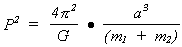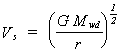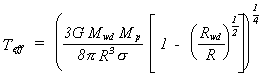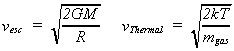Back to Assignments

A100 -- Dan Swearingen

Math Practice Homework - Points earned on this assignment will be applied towards any points lost on the In Class math quiz. Due Tuesday, September 24 at the beginning of class.

Numerical Problems:

Show all work.

Do all work on your own paper.

All answers must be in proper scientific notation: "n.mmm... x 10p".

Evaluate the following

1. 106 x 102 =

2. 1011/104 =

3. (4 x 10-6)/(8 x 10-4) =

4. 1/(100 x 1055 x 10-16 x 10 x 1082) =

5. 2 x 10-5 - 4 x 10-7 =

6. 3 x 1027 + 5 x 1028 =

7. 2 x 10-125 x 5 x 10122 =

8. (10 x 2 x 10-4 ) / (3 x 102 x 4 x 102 ) =

9. (7.5 x 10-9) / (3 x 10-9) =

10. (2 x 102A x 6 x 10-2L ) / (3 x 1012A x 7 x 104L ) =

11. 1018 + (3 x 1021) + (7 x 1024) =

For the following problems, solve for the variable indicated.

12. If:what is m1 = ?

13. If:what is Mwd ?

14. If:what is Mwd ?

Perform the following calculations:

The escape velocity vesc and the thermal velocity vThermal are given by the formulas:Calculate vesc and vThermal.

Where:

G = 6.7 x 10-11 [m3 kg-1 sec-2 ]

M = Mass of Earth = 5.9742 x 1027 kg

R = Radius of Earth = 6,378.14 km

k = 1.38 x 10-23 [J/oK]

mgas = mass of four protons = 4 x 1.67 x 10-27 kg

T = room temperature in Kelvin which is about 300o K

Note: make sure units are consistent. Answers should be velocities (a.k.a. speed) so final units should be in meters per second.